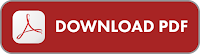## KTU B.TECH S3 THERMODYNAMICS BY P K NAG

Chapter-1: Introduction

Chapter-2: Temperature

Chapter-3: Work and Heat Transfer

Chapter-4: First Law of Thermodynamics

Chapter-5: First Law Applied to Flow Process

Chapter-6: Second Law of Thermodynamics

Chapter-7: Entropy

Chapter-8: Availability & Irreversibility

Chapter-9: Properties of Pure Substances

Chapter-10: Properties of Gases and Gas Mixture

Chapter-11: Thermodynamic Relations

Chapter-12: Vapour Power Cycles

Chapter-13: Gas Power Cycles

Chapter-14: Refrigeration Cycles

### Some Important Notes

•  Microscopic thermodynamics or statistical thermodynamics.
•  Macroscopic thermodynamics or classical thermodynamics.
• A quasi-static process is also called a reversible process.

Intensive and Extensive Properties

Intensive property: Whose value is independent of the size or extent i.e. mass of the system.

e.g., pressure p and temperature T.

Extensive property: Whose value depends on the size or extent i.e. mass of the system (upper case letters as the symbols).

e.g., Volume, Mass (V, M).

If mass is increased, the value of extensive property also increases.

e.g., volume V, internal energy U, enthalpy H, entropy S, etc.

Specific property: It is a special case of an intensive property.

It is the value of an extensive property per unit mass of system. (Lower case letters as symbols) e.g: specific volume, density (v, ρ).

CLICK HERE TO